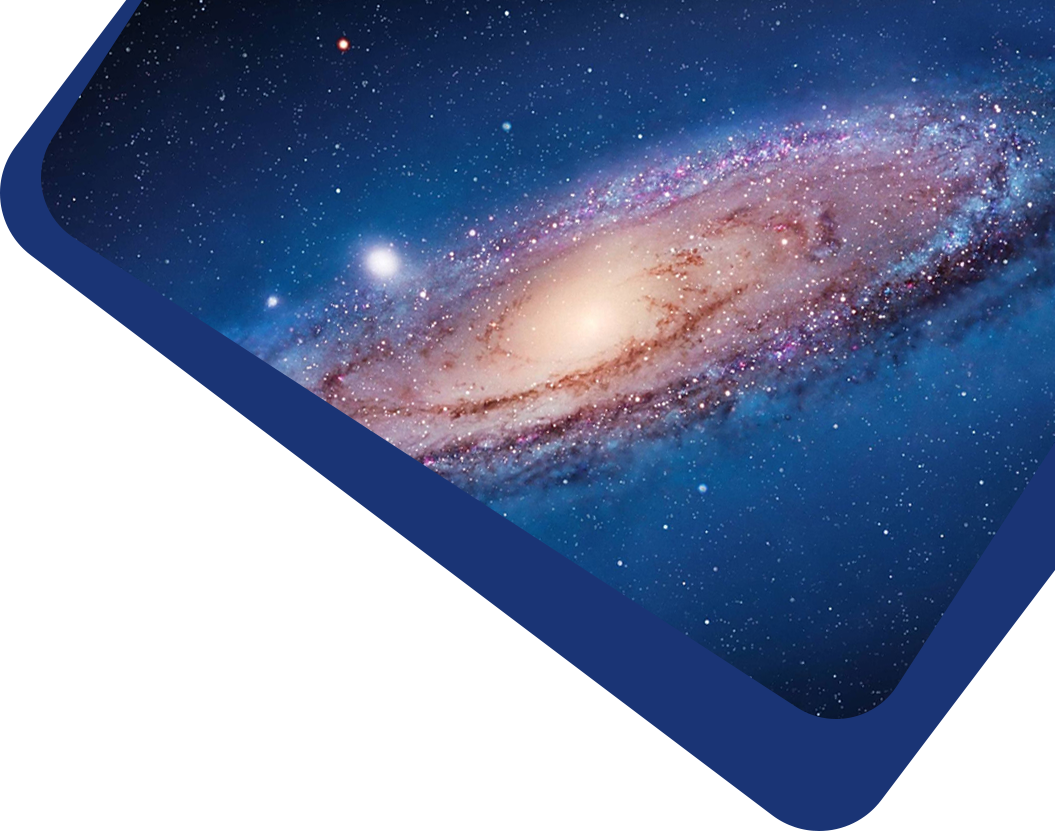Vol 21, No 9

## A strange star scenario for the formation of eccentric millisecond pulsar PSR J1946+3417

#### Long Jiang, Na Wang, Wen-Cong Chen, Wei-Min Liu, Chun-Wei Leng, Jian-Ping Yuan, Xiang-Li Qian

Abstract

Abstract PSR J1946 + 3417 is a millisecond pulsar (MSP) with a spin period $$P \simeq 3.17$$ ms. Harbored in a binary with an orbital period $$P_{\rm b}\simeq 27$$ days, the MSP is accompanied by a white dwarf (WD). The masses of the MSP and the WD were determined to be 1.83 $$M_{\odot}$$ and 0.266 $$M_{\odot}$$, respectively. Specially, its orbital eccentricity is $$e \simeq 0.134$$, which is challenging the recycling model of MSPs. Assuming that the neutron star in a binary may collapse to a strange star when its mass reaches a critical limit, we propose a phase transition (PT) scenario to account for the origin of the system. The sudden mass loss and the kick induced by asymmetric collapse during the PT may result in the orbital eccentricity. If the PT event takes place after the mass transfer ceases, the eccentric orbit cannot be re-circularized in the Hubble time. Aiming at the masses of both components, we simulate the evolution of the progenitor of PSR J1946 + 3417 via MESA. The simulations show that an NS / main sequence star binary with initial masses of 1.4 + 1.6 $$M_{\odot}$$ in an initial orbit of 2.59 days will evolve into a binary consisting of a 2.0 $$M_{\odot}$$ MSP and a 0.27 $$M_{\odot}$$ WD in an orbit of ∼ 21.5 days. Assuming that the gravitational mass loss fraction during PT is 10%, we simulate the effect of PT via the kick program of BSE with a velocity of $$\sigma_{\rm PT} = 60 \rm km~s^{-1}$$. The results show that the PT scenario can reproduce the observed orbital period and eccentricity with higher probability than other values.

Keywords

Keywords stars: neutron — stars: evolution — pulsars: individual (J1946+3417)

Full Text
Refbacks

• There are currently no refbacks.